Peshekhonov A.N.

Russia, РЎР°РјР°СҖР°, Samara state technical university, nova@mail.samtel.ru

PIECEWISE LINEAR APPROXIMATION OF NONLINEAR DYNAMIC SYSTEMS AT PRESENCE OF HINDRANCES IN OUTPUT SIGNALS

The known method of piecewise linear approximation is applied for identification of nonlinear dynamic models. However, the basic attention is given to a question of reception of consistent ratings for a case of presence autocorrelation noise in the form martingale differences, for models described with linear different equations. The original generalized method of the least squares is applied , it helps to receive consistent ratings of parameters of linear difference equations under weak restrictions on the input signal and hindrance.

РҹРөСҲРөС…РҫРҪРҫРІ Рҗ.Рқ.

Р РҫСҒСҒРёСҸ, Рі. РЎР°РјР°СҖР°, РЎР°РјР°СҖСҒРәРёР№ РіРҫСҒСғРҙР°СҖСҒСӮРІРөРҪРҪСӢР№ СӮРөС…РҪРёСҮРөСҒРәРёР№ СғРҪРёРІРөСҖСҒРёСӮРөСӮ, nova@mail.samtel.ru

РҡРЈРЎРһР§РқРһ-РӣРҳРқР•РҷРқРҗРҜ РҗРҹРҹР РһРҡРЎРҳРңРҗРҰРҳРҜ РқР•РӣРҳРқР•РҷРқР«РҘ Р”РҳРқРҗРңРҳР§Р•РЎРҡРҳРҘ РЎРҳРЎРўР•Рң РҹР Рҳ РқРҗРӣРҳР§РҳРҳ РҹРһРңР•РҘ Р’ Р’Р«РҘРһР”РқР«РҘ РЎРҳР“РқРҗРӣРҗРҘ

Р”Р»СҸ РёРҙРөРҪСӮРёС„РёРәР°СҶРёРё РҪРөР»РёРҪРөР№РҪСӢС… РҙРёРҪР°РјРёСҮРөСҒРәРёС… РјРҫРҙРөР»РөР№ РҝСҖРёРјРөРҪРөРҪ РёР·РІРөСҒСӮРҪСӢР№ РјРөСӮРҫРҙ РәСғСҒРҫСҮРҪРҫ-Р»РёРҪРөР№РҪРҫР№ Р°РҝРҝСҖРҫРәСҒРёРјР°СҶРёРё. РһРҙРҪР°РәРҫ, РҫСҒРҪРҫРІРҪРҫРө РІРҪРёРјР°РҪРёРө СғРҙРөР»СҸРөСӮСҒСҸ РІРҫРҝСҖРҫСҒСғ РҝРҫР»СғСҮРөРҪРёСҸ СҒРҫСҒСӮРҫСҸСӮРөР»СҢРҪСӢС… РҫСҶРөРҪРҫРә РҙР»СҸ СҒР»СғСҮР°СҸ РҪР°Р»РёСҮРёСҸ Р°РІСӮРҫРәРҫСҖСҖРөР»РёСҖРҫРІР°РҪРҪРҫРіРҫ СҲСғРјР° РІ С„РҫСҖРјРө РјР°СҖСӮРёРҪРіР°Р» СҖР°Р·РҪРҫСҒСӮРөР№, РҙР»СҸ РјРҫРҙРөР»РөР№, РҫРҝРёСҒСӢРІР°РөРјСӢС… Р»РёРҪРөР№РҪСӢРјРё СҖР°Р·РҪРҫСҒСӮРҪСӢРјРё СғСҖР°РІРҪРөРҪРёСҸРјРё. РҹСҖРёРјРөРҪРөРҪ РҫСҖРёРіРёРҪР°Р»СҢРҪСӢР№ РҫРұРҫРұСүРөРҪРҪСӢР№ РјРөСӮРҫРҙ РҪР°РёРјРөРҪСҢСҲРёС… РәРІР°РҙСҖР°СӮРҫРІ, СҒ РҝРҫРјРҫСүСҢСҺ РәРҫСӮРҫСҖРҫРіРҫ РҝСҖРё СҒР»Р°РұСӢС… РҫРіСҖР°РҪРёСҮРөРҪРёСҸС… РҪР° РІС…РҫРҙРҪРҫР№ СҒРёРіРҪР°Р» Рё РҝРҫРјРөС…Сғ СғРҙР°РөСӮСҒСҸ РҝРҫР»СғСҮРёСӮСҢ СҒРҫСҒСӮРҫСҸСӮРөР»СҢРҪСӢРө РҫСҶРөРҪРәРё РҝР°СҖР°РјРөСӮСҖРҫРІ Р»РёРҪРөР№РҪРҫРіРҫ СҖР°Р·РҪРҫСҒСӮРҪРҫРіРҫ СғСҖР°РІРҪРөРҪРёСҸ.

The report is concerned with regression models, which though are nonlinear functions within a whole allowable area of change of the input variables, but the approximation is possible with their linear functions within the limits of separate sub areas of input space.

The basic feature of each of sub areas of change of the input variables is the availability of auto correlated noise in the observation channel, the model is being set in the form of linear difference equation.

Description of dynamic objects with linear difference equations depends on a point of application of a hindrance. A hindrance is being available at the input of the direct channel (before the operator of a feedback channel of use of usual procedure of method of least squares (РңLS) allows to receive strongly consistent estimates under rather weak restrictions to an input signal and hindrance. But in case an additive hindrance is applied in the output channel of object the task of identification becomes already the task of regression analysis with mistakes in independent variables; thus the estimates of parameters received with usual MLS will be inconsistent , even if a hindrance is a stationary discrete white noise.

The report describes the algorithm of nonlinear РңLS for identification of parameters of linear difference equations under additive locally auto correlated noise in the observation channel . The strong consistency of offered estimates is proved under conditions to an input signal and hindrance. The questions of existence of these estimates are being considered . The proof of strong consistency is based upon the theorem of convergence of martingale and KroneckerвҖҷ s lemma .

Let us consider the stationary dynamic system, described with the following linear difference equation of the given order with discrete time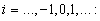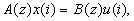(1)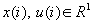and output variable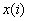is observed with additive noise as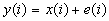(2)

where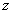- operator of delay;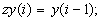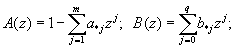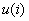and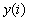- observable input and output signals accordingly;- unobservable output signal;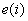- unobservable noise;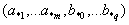- unknown material true value of parameters.

Basing upon observable final realizations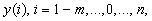and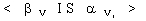it is required to determine estimates of unknown true value of parametersunder the following assumptions:

1). The stochastic processsatisfies the conditions: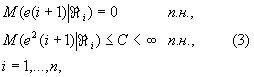where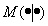- conditional mathematical expectation;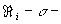algebra, induced by the class of random variables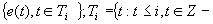set of integers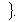2). The following conditions are met :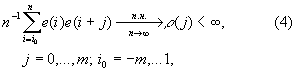where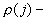local autocovariation function.

Matrix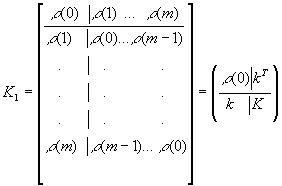Positively determined, where СӮ- transposition mark, and matrix K: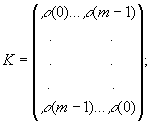vector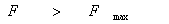.

3). Random variablesdoes not depend upon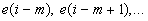4). The true value of parameters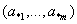satisfy stationary state conditions, i.e. roots of the characteristic equation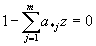lay outside a unit disk.

5). The input signalis a random process and satisfies the conditions of constant excitation of the order q, i.e. with probability 1 exists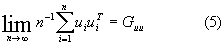and matrix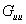positively determined, where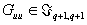- set of rectangular material matrixes of dimension (q+1)пӮ·(q+1);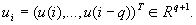Let a stationary dynamic system is described by the equations (1), (2) and the hindrance satisfies the assumptions (3) - (5).

Then at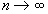with probability 1 there is an estimate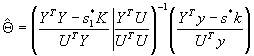where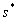- the least root of the equation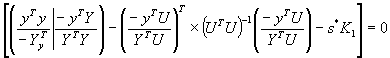and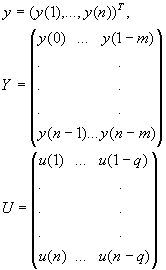thus the estimation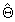- is strong solvent. In the report are given the software and tests.

 Site of Information Technologies Designed by  inftech@webservis.ru.